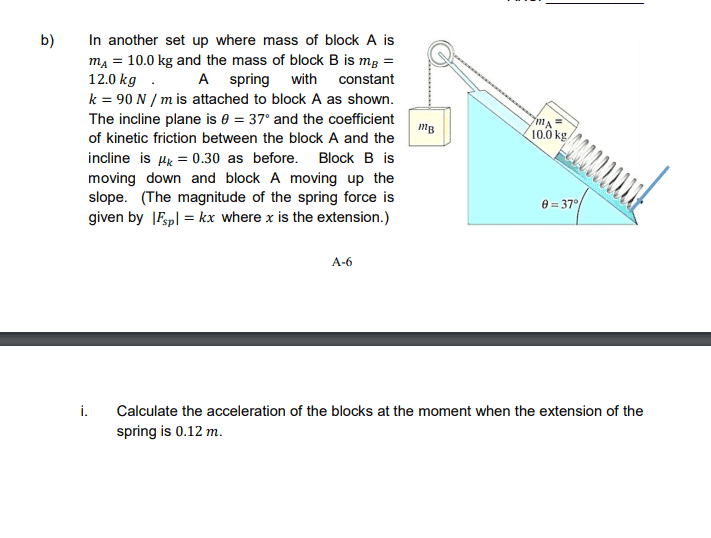nicky670
Homework Statement:
In another set up where mass of block A is 𝑚𝐴 = 10.0 kg and the mass of block B is 𝑚𝐵 =12.0 𝑘𝑔. A spring with constant𝑘 = 90 𝑁 / 𝑚 is attached to block A as shown. The incline plane is 𝜃 = 37∘ and the coefficient of kinetic friction between the block A and theincline is 𝜇𝑘 = 0.30 as before. Block B is moving down and block A moving up the slope. (The magnitude of the spring force is
given by |𝐹𝑠𝑝| = 𝑘𝑥 where 𝑥 is the extension.)
Relevant Equations:
For Ma(perpendicular) = FN - Magcos(titre) = 0
Ma (Parallel) = Fsp + T - Ffriction - Magsin(titre) = Ma(a)

For Mb: Mbg - T = Mb(a)
Fsp = 90 x 0.12 = 10.8
Ffriction = Magcos(titre) x 0.30
I got the answer 2.09ms2 when the correct answer is 1.11ms2. What am i doing wrong here?Last edited by a moderator:

Mentor
Isn't the spring force opposite to T?

nicky670
Isn't the spring force opposite to T?
But wouldn't the spring force push the block forward?

Homework Helper
Gold Member
2022 Award
But wouldn't the spring force push the block forward?
It says the spring has an extension of 0.12m.

nicky670
It says the spring has an extension of 0.12m.
When the spring extends, wouldn't it exert a force in the same direction as the tension force?

Mentor
When the spring extends, wouldn't it exert a force in the same direction as the tension force?
When you stretch a spring, doesn't it pull back on you in the opposite direction?

Homework Helper
Gold Member
2022 Award
When the spring extends, wouldn't it exert a force in the same direction as the tension force?
Is an extended spring under tension or under compression?

nicky670
When you stretch a spring, doesn't it pull back on you in the opposite direction?
Oh, means to say when its extended it will have a tension pulling it back and if compressed there will be a force pushing the block forward?

nicky670
Is an extended spring under tension or under compression?
tension.